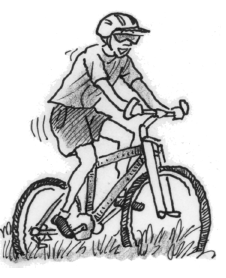### Home > APCALC > Chapter 6 > Lesson 6.3.1 > Problem6-87

6-87.

While riding his bike to a lake, Steven’s distance in miles is modeled by $s(t) = t^3 - 3t^2 + 3t$. If the lake is $9$ miles away and $t$ is measured in hours:

1. What was Steven’s maximum velocity during the trip? When did it occur?

Visualize $v(t)$. It is a concave up parabola; therefore there cannot be a local maximum. But Steven didn't ride his bicycle forever! You can still find the maximum velocity during the time interval when Steven was on his bike... Check the Endpoints!

$v(t) = 3t^2 − 6t + 3 = 3(t − 1)^2$

2. Did Steven ever stop during the trip? Justify your conclusion analytically.

Does $v(t)$ ever equal $0$ during the time interval of Steven's bike ride?

3. What was Steven’s average velocity?

$\text{average velocity }=\frac{\text{total displacement}}{\text{total time}}$.